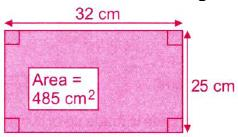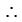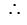Courses

# The shaded part in the given figure is covered with cement. If it costs ` 84 to: cement an area of 23 cm , find the total cost of cementing.a)Rs. 8820b)Rs. 8125c)Rs. 8610d)Rs.8804Correct answer is option 'A'. Can you explain this answer? Related Test: Test : Perimeter And Area - 2

## Class 5 Question

By PRASOON KUMAR · Aug 29, 2020 ·Class 5
SOUMYA DAS answered Aug 30, 2019
Area of larger rectangle
= (32x25) cm2 = 800cm2
Also, area of smaller rectangle = 485 cm2= (800 - 485) cm = 315cm2
Now,
Cost of cementing of 3cm2 = Rs.84Cost of cementing of 1 cm2
= (84/3)
= Rs.28Cost of cementing of 315 cm2
= Rs. (28x315) = Rs.8820